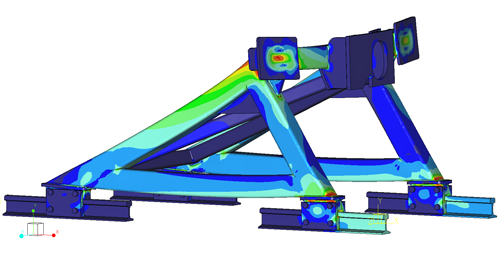# Strength analysis / stiffness analysis / FEM analysis

## Determining strength

The strength and stiffness of a product or construction can be determined and interpreted in different ways. In general the calculated stresses due to forces that are put on a construction are compared to the allowable stresses for the materials of which the construction is made. If the analysed stresses are lower than the allowable stresses the construction is strong enough. So the allowable material stress values are very important for the strength calculation.

## Analysing methods

For simple constructions the strength can be calculated by hand. More complex constructions require the Finite Element Method (FEM) or Finite Element Analysis (FEA) where the complex part is divided in a finite number of elements of which the material properties are known.To get an accurate result the distribution of the elements (mesh) needs to be fine enough. By refining the mesh step-by-step and analysing the results this can be determined. This can be done by hand, but is mostly done by using FEM software.

## Norms

As stated above the allowable stresses are very important for the strength analysis. Many constructions need to comply to norms. These norms mostly define the allowable stresses, often a factor of the yield strength or tensile strength of the given material. However many of these norms are written for the calculation by hand and therefore have a low accuracy. If this data is used in a FEM calculation there is a high chance that you have to make the construction bigger than necessary. You don’t want that of course.
Luckily, nowadays norms exists that are specially written for the use with FEM analysis. Examples are: ASME VIII Div.2 and EN 13445. This is why, if possible, we like to use this data to analyse the strength (it is originally written for pressure valves, but reasonably universally applicable). These norms have a number of stress categories with their own allowable stress. Very local peak stresses even don’t have to comply to the allowable stress, but are just analysed for durability.

## Plasticity

There is another way to determine the strength: plastic strain. In some heavily loaded constructions (especially welded constructions) there are always stresses above the yield strength of the material. A re-distribution of the stresses occurs by plastic deformation, which causes the construction to hold in practice. This is why the norms offer analysis based on plastic strains. This requires a non-linear FEM calculation with a plastic material behaviour to simulate the plastic deformity in the construction. The maximum plastic strain is compared to the tensile strength of the material.